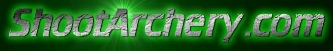ODPS Dimensions for Success
Front of Center Calculator
You will need IE4 and above or Netscape 4.08 and above to use

Percent Front Of Center, represents the percentage of total arrow weight that is located in the front half of the arrow. The amount of FOC needed, depends on the type of archery that will be shot with that particular arrow. There is no perfect F.O.C. for each setup.
But here are examples of some good
starting points.
To calculate %FOC, you will need to know two dimensions:
1. Total Length (L) - Total arrow length in decimal inches. (ie: 27.5).
2. Nock to Balance Point (A) - The distance in decimal inches from
the Nock to the point on the arrow shaft where it will balance.
(includes weight of point plus insert, nock system and fletching)
 FITA 3-D Field Hunting 11-16%   6-12% 10-15% 10-15%AMO-Standard F.O.C. balance formula: %FOC = (100x(A-L/2))/L
Total Length (L)
Nock to Balance Point (A)

Be sure to check out:
Ke Calculator | %Let off Calculator | back to ShootArchery.com